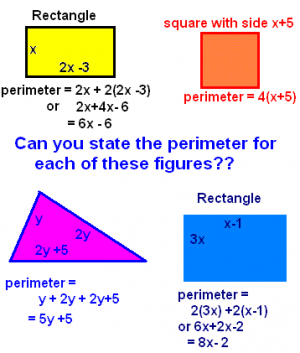# Perimeter of triangles, squares and more

Posted on: March 21, 2020 Posted by: Susan Johnsey Comments: 0Find the length of a rectangle whose perimeter is 40 feet.  The length is 2 more than 5 times the width.     What is the length if we let the width be w? Then the length is 2 more than 5 times w.  That is 2 +5w or 5w + 2.  The perimeter is 2 widths added to 2 lengths:  P = w+ w+ 5w+2 + 5w+2 We know P is 40. SEE that in the stated problem above.

40 = 12w + 4          Now solve for w.   36 = 12w thus   w = 3 feet  and the length is 5(3) + 2= 17.

Check the perimeter by adding the 2 widths and  2 lengths.

3+3+17+17 = 40 feet.